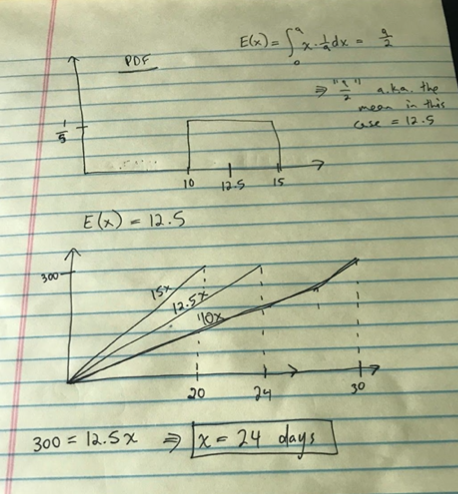# JUNTO Practice: Probability, Lemonade Stand

Discussed on May 05, 2020.

Problem statement:

I make between \$10 and \$15 a day running my lemonade stand. What is the expected numbers of days that it will take for me to make \$300?

## Solutions

Click to see:

### Oscar Martinez

``````#!/usr/bin/env python

import numpy as np

# We can assume either that our stand makes \$10 or \$15 with equal probability (0.5)
# Or we can assume a uniform distribution U~(10,15)
# In any case the answer is the same
probability_10 = 0.5
probability_15 = 0.5
daily_expected_revenue = (probability_10 * 10) + (probability_15 * 15)
print(f"Daily Expected Revenue: \${daily_expected_revenue}")

expected_days_till_300 = 300 / daily_expected_revenue
print(f"Expected Days to \$300: {expected_days_till_300} days")

# Now let's try sampling from a uniform distribution
# We can prove E(U~(a,b)) = (b + a) / 2, but let's show it by randomly sampling
np.random.seed(777)
revenue_distribution_samples = np.random.uniform(low=10.0,high=15.0,size=100_000_000)
mean_revenue = np.mean(revenue_distribution_samples)
print(f"Daily Expected Revenue: \${mean_revenue}")

u_expected_days_till_300 = 300 / mean_revenue
print(f"Expected Days to \$300: {u_expected_days_till_300} days")
``````
``````Daily Expected Revenue: \$12.5
Expected Days to \$300: 24.0 days
Daily Expected Revenue: \$12.499969938379195
Expected Days to \$300: 24.000057718450755 days
``````

### John Lekberg

I expect that it will take 25 days to earn at least \$300.

• I created a function in Python to simulate running the lemonade stand.
• I ran the simulation 10,000 times to generate a random sample.
• I took the mean of the sample and rounded it up (because I have to work a whole number of days).
``````import math
import random
import statistics

def random_daily_profit():
"""Sample the random variable "one day's profit", which
is modelled using a continuous uniform distribution
between \$10 and \$15.
"""
return random.uniform(10, 15)

def random_days_to_300():
"""Sample the random variable "days to make \$300", which
is modelled by a random walk in which each step is
sampled from the random variable "one day's profit".
"""
profit = 0
days = 0

while profit <= 300:
days += 1
profit += random_daily_profit()

return days

N = 10_000
sample = [ random_days_to_300() for _ in range(N) ]

math.ceil(statistics.mean(sample))
``````
``````25
``````

### Daniel Bassett

Assumption: The day's payment is a continuous random variable with probability 1/a.

Because each day’s payment occurs with equal probability 1/a, the probability of each day’s payment creates a Probability Density Function in the shape of a rectangle. We can then assume Expected Values (E(x)) to find the mean of the day’s payment variable and then solve to find the number of days from that expected value.